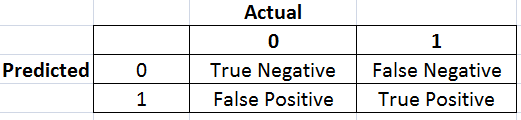## Sunday, February 09, 2014

### Calculate Confusion Matrix Using SQL

#### Problem

Confusion matrix is often used to measure the accuracy of a predictive model. For example, for a model that predicts binary outcomes, if we compare the prediction and actual outcome, say 0 or 1, there are four possibilities: true positive (predicted 1 and actual 1), false positive (predicted 1 and actual 0), true negative (predicted 0 and actual 0) and false negative (predicted 0 and actual 1)as shown in the diagram below.How to calculate the confusion matrix using SQL?

#### Solution

We can easily calculate the confusion matrix using SQL "group by". For example, the following query first applies model GLM1031E to data set DATASET_TEST using prediction() function. Then "group by" is used to calculate the number of records for all combinations of predicted and actual outcomes.

```SQL> with tbl as (select prediction(GLM1031E using * ) predicted,
account_default as actual from DATASET_TEST) select predicted, actual,
count(1)
from tbl group by predicted, actual;

PREDICTED     ACTUAL   COUNT(1)
---------- ---------- ----------
1          0          3
0          0        437
1          1         41
0          1          3
```
I have found that in real world application people pay a great deal of attention to false positive. For example, in credit card fraud detection, there are only a few fraudulent transactions out of ten thousand. If our predictive model can detect correctly one true frauds (true positive) while make three false alarms (false positive), it is considered pretty good.